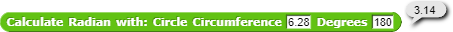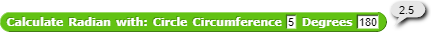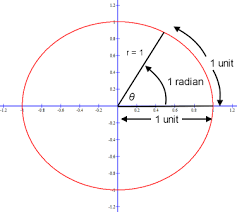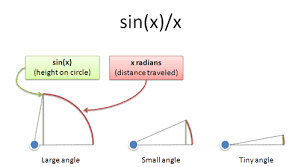What's the difference between Radians and Degrees?

Degrees measures the angle between one point and another point of a circle. Radians measures how long it is one point to another point of a circle.

For anyone who likes Radians or wants to learn about them, here's a project filled with blocks which can calculate Radians and convert Radians to Degrees.

No, radians is an unit-less angle measure. Can be expressed as (arc len)/circumference.

So only the result for the circle with radius =1>> circ.= 2*$$\pi$$ is equal to radiansIn any other case it's only arc len for the given angle.I'm guessing you mean that radians don't involve the unit of distance that you choose, but that's true of any angle measurement. But radians are a unit of angle, just as degrees are a unit of angle. That's why it makes sense to talk about converting between radians and degrees.

I think this is a confusing way to put it. Radians and degrees are both angle measurements, not distance measurements. The radian is chosen as a preferred angle measurement because it's a ratio of distances, and it works as an angle measurement because all circles are similar, just as all parabolas are similar.

But there's no obvious reason why using the ratio of arc length to radius is a good way to measure angles. In practical terms, degrees are much better, because all the angles you care about become integers rather than being irrational. (This is why even Europeans measure angles in degrees.) Radians don't have any particular virtues until you get to calculus and, more specifically, into Fourier series and functions of complex numbers. In that context they make the arithmetic easier.

I don't known the proper wordings: The radian is defined in the SI as being a dimensionless unit

But there's no obvious reason why using the ratio of arc length to radius is a good way to measure angles. In practical terms, degrees are much better, because all the angles you care about become integers rather than being irrational.

radians make arithmetic easier even in simpler contexts, because they're just a much more reasonable unit.
a good think to note about units is that you can treat them like any other variable in math.

for example, i can make a physics problem for displacement:
d=vt+a(t^2/2)
d=(20 cm/s)(5 s)+(1m/s^2)((5 s)^2/2)
d=(100 cm)+(1m)((25 s^2)/2) <--- yes, that's s^2 by itself
1m=100cm
d=(1m)+(1m/s^2)(25/2 s^2)
d=(1m)+(25/2 m)
d=27/2 m

the reason acceleration is distance/time^2 is just because that's how the math works. i'm not sure what exactly square time is, but if you can square distance, why not?

let's find some equation with units that would use an angle. here's one:
radius is used here since that's generally a lot more useful in equations.
note here that the radius of a circle is still just a distance!
distance=(angle)(distance)
distance/distance=angle
1=angle
the unit of an angle is simply 1. it generally makes more sense to think of the unit as an amount.
one radian is the angle created from taking the length of the radius and going that far around the circle. this standard unit is called a radian. rad = 1

if you don't like this unit, we can create another!
how about a turn? it's simply the angle around a circle to get back to the start.
the symbol for a turn is 𝜏. however, we still did that math before, 𝜏 is a known value. it's still just a number and can be simplified (as in, you can follow instructions to simplify it. you would never actually finish).
both turns and radians are great units that can be interchanged depending on the situation. for example, with a radian, you can measure distance traveled around, letting you measure movement from wheels and gears. turns are great for trigonometry. both are quite intuitive.

there's also the half-turn, denoted with π. again, it's a number, and is awkward anyways as it's half a turn. it exists for compatibility with legacy textbooks. because it's half, 2 and 1/2 has to get thrown around all the math.
there's also the degree, with the symbol °. it's 1/360th of a turn. why 360? now the number has to get thrown around everywhere just like the half-turn.
there's also the gradian, written as gon. it's equal to 1/400th of a turn. this is a terrible unit.

Yes, I misspoke. In problems that are about distances, angles are considered dimensionless. But, let's pose the problem "Through what angle does the turtle have to turn at each vertex in order to make a regular pentagon?" And the answer is

turning = circle/(number of vertices)

So, what's "circle"? It's the amount the turtle has to turn in order to get back to its initial heading, so you can think of it as 360°, or 𝜋/2 radians, etc. And those are values with units.

(In this problem the number 5, which represents the number of vertices, is unitless.)

But my point was that it doesn't make sense to talk about degrees and radians as if one were a unit and the other a pure number. Degrees and radians have the same status as a unit of angle, i.e., a unit of turning.

The fight about 𝜋 vs. 𝜏, about which there are maybe six people in the world who care, is orthogonal to the question of whether angles are measured in units. And the answer to that has to be yes; otherwise you have to say that an answer in degrees is wrong, which is overstating the case.

P.S. When you say that under a constant acceleration, such as the acceleration due to gravity, the distance is ½at² you are doing calculus.

2TT

There are about 9.42 people who care,about 6.28 think that pi is better,about 3.14 think that tau is better,and about 0.58 think that full turns are better,and im that 0.58+that 0.28+ that 0.14
/j

Yeah.
Specifically,solving a Linear Second-order Homogenous Seperatable Asbestos-Free Differential Equation
(sorry if i got some of the big words wrong,because i did not listen to calculus class carefully because there weren't any yet(sixth grade))

rereading my previous post, there is some issues, i'll try to clarify now
units are variables that specify what numbers mean. 1 rad = 1, but the 1 here doesn't have a unit, while rad indicates an angle

this unit is the turn i mentioned earlier, with the symbol 𝜏. 𝜏 = 2π, not π/2
i never called anything a "pure unit or number", any number or variable can be a unit. it's like saying a "subject or noun", it depends on the context and one is the other. the reason that a radian = 1 is because that's just how the units work out when doing math to them.
if i give your equation units:
turning(angle) = 𝜏(angle) / vertices(amount)
we can consider this dividing an angle by an amount to get an angle, or dividing a number by a number to get a number. the latter is just less descriptive, not giving any information about what the result actually is.

you can multiply an area by an amount to get that many of the area, but if you multiply an area by an angle, i'm not sure what that would mean. polar area? the use case would be different.

it does have a unit, amount. it measures how much of something there is. an angle is an amount of radius. if a number didn't have a unit, it might not even mean an amount, it could be anything.

𝜏 is equal to one turn, or twice the area of a unit circle. 𝜋 is equal to half a turn, or the area of a unit circle. i used them appropriately, and it's very clear that for measuring angles, a turn is generally more useful than half a turn. when measuring small objects, it's easier to use cm than m, and that doesn't mean there's a fight between cm and m.

the only person here that said "unit-less" immediately said they don't know the proper wording and clarified with a link to dimensionless unit. i don't know what would give you the impression that i thought angles weren't a unit when i said multiple times that they are, and that degrees are too.
° = 𝜏/360. degrees are simply annoying to use because you need to constantly throw around 360, since that number comes out of nowhere and doesn't relate to anything useful.

where's the calculus? i did the equation with only basic algebra.
even if it was calculus, that doesn't seem at all relevant because that's not where i used angles, and i never involved calculus anywhere near angles.

So you did
s=at
d=st
so substitution d=at^2?
THATS WRONG
you need integrals

read the post? that is clearly not what i did.

bh meant the derivation

Okay, this is getting to be a silly thing for us to argue about, but if you're doing dimensional analysis and you end up with an equation such as "1 rad = 1" that's an error. One radian and dimensionless one are incommensurable!

The point for our purposes is that SI units were defined for the convenience of engineers, not of mathematicians. Engineers would never measure angles in turns, because that's too big a unit to be practical. Instead they'd end up using the deciturn, one tenth of a turn, just as they measure sound pressure in decibels or, for that matter, distances in centimeters. (This is also why we reactionaries continue to measure temperature in °F instead of °C, which is too big a unit to be practical in trying to decide what to wear.)

how far does a 1 meter radius wheel travel if rotated 1 radian?
note that the distance can just be canceled out
can a radian be used for something other than comparing distances? i'm not sure, but the unit is still dimensionless.

sourcing via brute force? wikipedia has a lot of links.
i don't feel like you quoted enough that i understand what you're trying to say with it. it doesn't say anything about engineering, who huntley is, what their idea is, or if their idea is standard.

where did you ever say engineers? everything everyone here has talked about is just math. don't engineers need to do math anyways too? they make wheels and gears and such.

another unit! now 10 needs to be thrown around everywhere, and dividing a turn up into thirds, sevenths, ninths, etc still isn't practical. there's still nothing stopping it from being used though, it's just still inconvenient.
although, i notice that i can't find the word "deciturn" on wikipedia, or the rest of the internet. if this is what engineers use, why can't i find it anywhere?

I don't even think that its a unit.
In english, you can say "2 radians" but in the calculation you just say sin(2) not sin(2 radians)
Contrast,degrees is actually a dimentionless unit,and its value is pi/180
sin(90°)=sin(pi/2)=1

idk,engineers actually use radians and sometimes turns but never degrees or deciturns or gradians felsius

Sorry, I meant to include the page reference but spaced out: orientational analysis.

The link didn't take me directly to the relevant paragraphs so you have to search within the page. I quoted from two different places in the article, separated by an ellipsis in my text.

Or you can just believe me about what it says: What counts as "dimensionless" depends on the problem you're trying to solve. In most engineering problems (e.g., which direction to point your cannon for the furthest range) it's convenient to treat angles as dimensionless, because what you want is to maximize a distance, and to do that you also have to think about velocity and acceleration.

It's to solve the problems that engineers care about that they developed SI units. Those units weren't handed down on tablets from God.

This:

is an engineering problem, not a (pure) math problem, although of course you use math to help solve it. All the "math" problems you've used as examples are about physics, and more specifically about mechanics, not about math.

About "deciturn," I thought the context made it clear that this isn't a unit used in practice, but neither is "turn." And if you started throwing turns around, rather than degrees or radians, engineers would say that 1 turn is too big a unit to be practical, and so they'd divide it by 10, as happens with other units that are too big to be practical, such as meters (to cm), bels (to decibels), Farads (to µF), and others I don't remember offhand. The resulting unit, 1/10 turn, would be called a deciturn to fit the standard SI practice of using powers of ten to get a unit down to a usable size.

I'm saying "engineer" rather than "physicist" because the latter aren't solving specific practical problem and therefore don't care if their units are enormous (or, in the case of quantum physics, tiny).